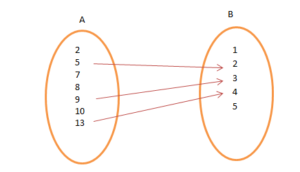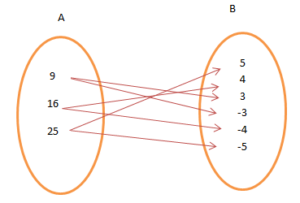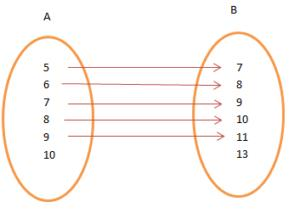Courses

# Introduction, Relations Mathematics Notes | EduRev

## Mathematics : Introduction, Relations Mathematics Notes | EduRev

The document Introduction, Relations Mathematics Notes | EduRev is a part of the Mathematics Course Algebra for IIT JAM Mathematics.
All you need of Mathematics at this link: Mathematics

Relation
Given any two non-empty sets A and B, A relation R from A to B is a subset of the Cartesian product A x B and is derived by describing a relationship between the first element (say x) and the other element (say y) of the ordered pairs in A & B.
Consider an example of two sets,  A = {2, 5, 7, 8, 9, 10, 13} and B = {1, 2, 3, 4, 5}. The Cartesian product A × B has 30 ordered pairs such as A × B = {(2, 3), (2, 5)…(10, 12)}. From this, we can obtain a subset of A × B, by introducing a relation R between the first element and the second element of the ordered pair (x, y) as
R = {(x, y): x = 4y – 3, x ∈ A and y ∈ B}
Then, R = {(5, 2), (9, 3), (13, 4)}.(Arrow representation of the Relation R)

Representation of Relation
A relation is represented either by Roster method or by Set-builder method. Consider an example of two sets A = {9, 16, 25} and B = {5, 4, 3, -3, -4, -5}. The relation is that the elements of A are the square of the elements of B.

• In set-builder form, R = {(x, y): x is the square of y, x ∈ A and y ∈ B}.
• In roster form, R = {(9, 3), (9, -3), (16, 4), (16, -4), (25, 5), (25, -5)}.Terminologies

• Before getting into details, let us get familiar with a few terms:
• Image: Suppose we are looking in a mirror. What do we see? An image or reflection. Similarly, for any ordered pairs, in any Cartesian product (say A × B), the second element is called the image of the first element.
• Domain: The set of all first elements of the ordered pairs in a relation R from a set A to a set B.
• Range: The set of all second elements in a relation R from a set A to a set B.
• Codomain: The whole set B. Range ⊆ Codomain.

Total Number of Relations

For two non-empty set, A and B. If the number of elements in A is h i.e., n(A) = h & that of B is k i.e., n(B) = k, then the number of ordered pair in the Cartesian product will be n(A × B) = hk. The total number of relations is 2hk.

Solved Examples for You

Problem: Let A = {5, 6, 7, 8, 9, 10} and B = {7, 8, 9, 10, 11, 13}. Define a relation R from A to B by
R = {(x, y): y = x + 2}. Write down the domain, codomain and range of R.
Solution: Here, R = {(5, 7), (6, 8), (7, 9), (8, 10), (9, 11)}.Domain = {5, 6, 7, 8, 9}
Range = {7, 8, 9, 10, 11}
Co-domain = {7, 8, 9, 10, 11, 13}.

Offer running on EduRev: Apply code STAYHOME200 to get INR 200 off on our premium plan EduRev Infinity!

58 docs

,

,

,

,

,

,

,

,

,

,

,

,

,

,

,

,

,

,

,

,

,

,

,

,

;